Скачать презентацию Multiplying and Dividing Greater Numbers 1 3 5

c924c6f8c9239ca016a671ad0b9a9722.ppt

• Количество слайдов: 44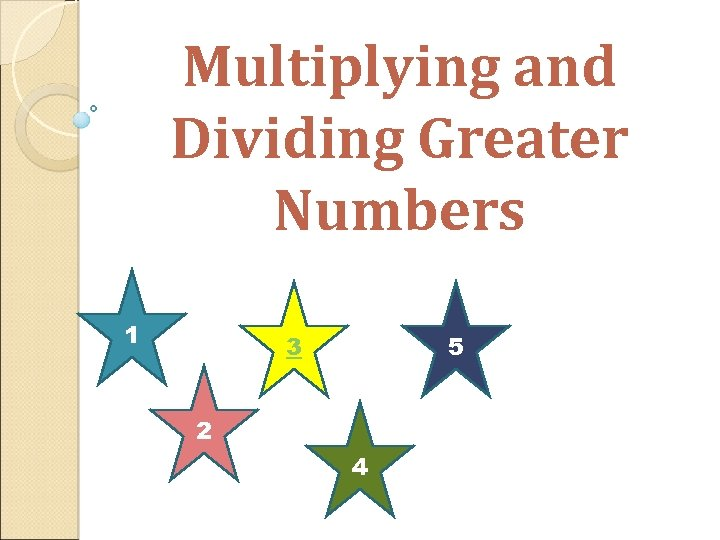Multiplying and Dividing Greater Numbers 1 3 5 2 4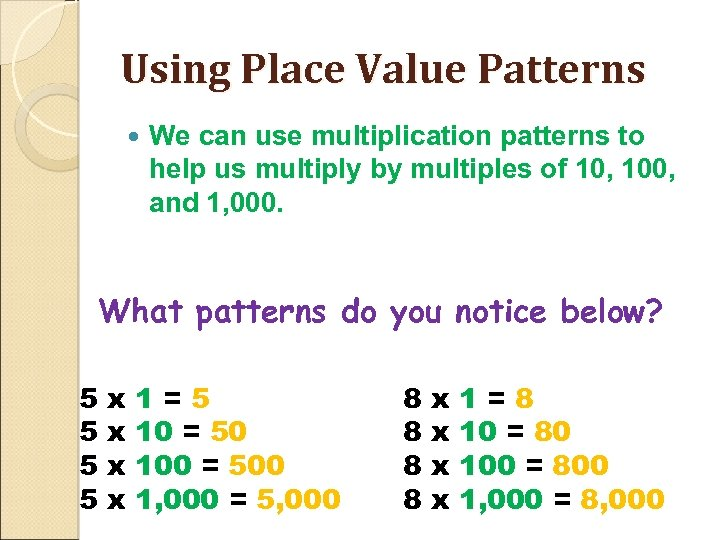Using Place Value Patterns We can use multiplication patterns to help us multiply by multiples of 10, 100, and 1, 000. What patterns do you notice below? 5 5 x x 1=5 10 = 50 100 = 500 1, 000 = 5, 000 8 8 x x 1=8 10 = 80 100 = 800 1, 000 = 8, 000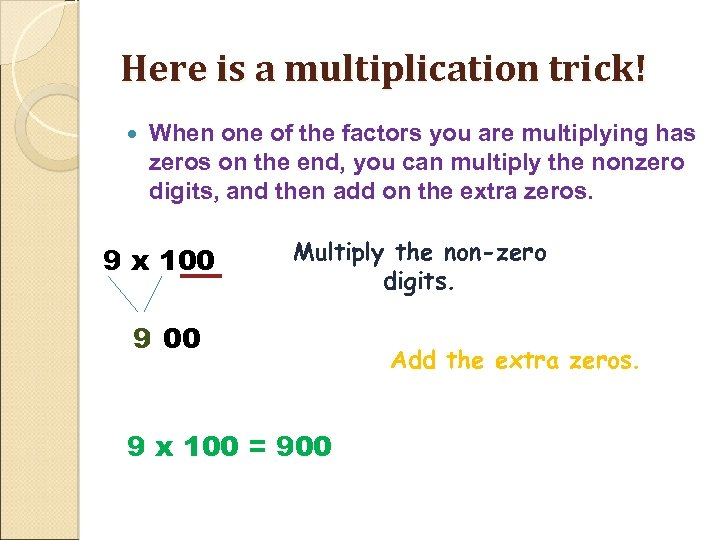Here is a multiplication trick! When one of the factors you are multiplying has zeros on the end, you can multiply the nonzero digits, and then add on the extra zeros. 9 x 100 Multiply the non-zero digits. 9 00 9 x 100 = 900 Add the extra zeros.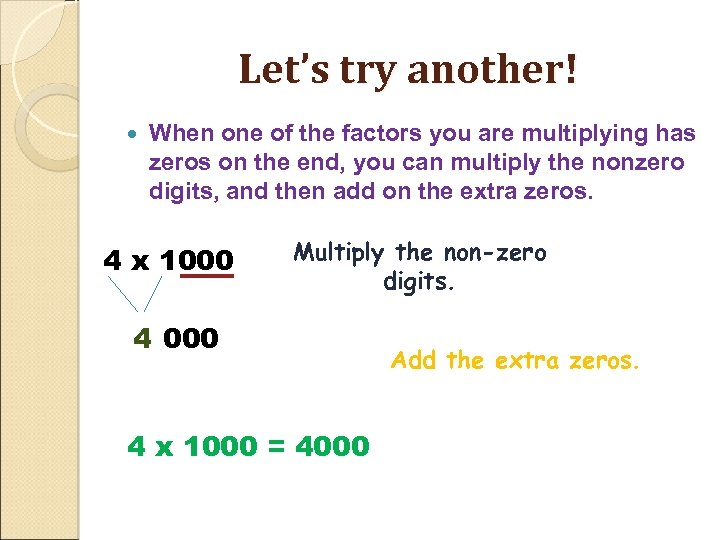Let’s try another! When one of the factors you are multiplying has zeros on the end, you can multiply the nonzero digits, and then add on the extra zeros. 4 x 1000 Multiply the non-zero digits. 4 000 4 x 1000 = 4000 Add the extra zeros.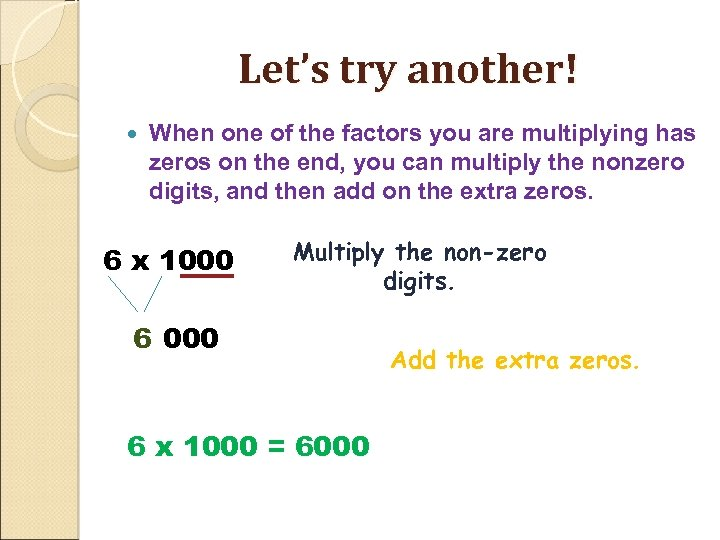Let’s try another! When one of the factors you are multiplying has zeros on the end, you can multiply the nonzero digits, and then add on the extra zeros. 6 x 1000 Multiply the non-zero digits. 6 000 6 x 1000 = 6000 Add the extra zeros.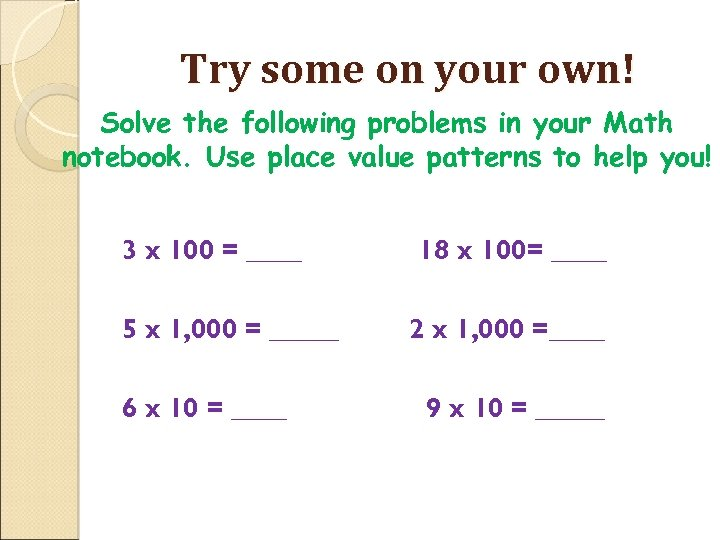Try some on your own! Solve the following problems in your Math notebook. Use place value patterns to help you! 3 x 100 = ____ 5 x 1, 000 = _____ 6 x 10 = ____ 18 x 100= ____ 2 x 1, 000 =____ 9 x 10 = _____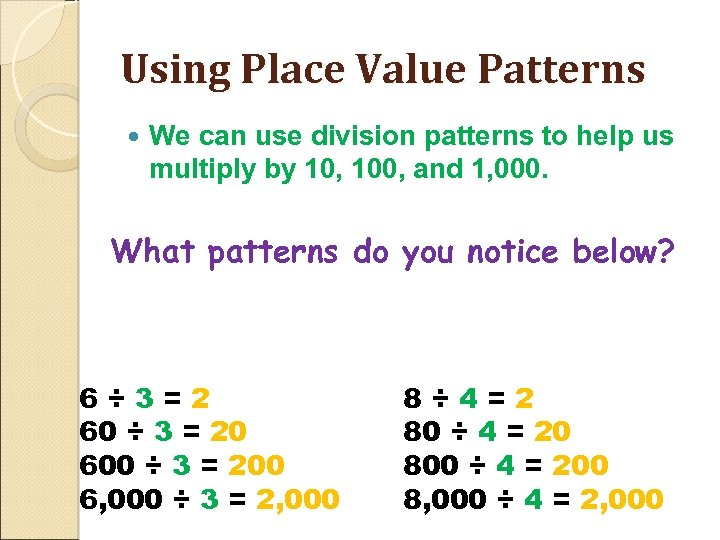Using Place Value Patterns We can use division patterns to help us multiply by 10, 100, and 1, 000. What patterns do you notice below? 6÷ 3=2 60 ÷ 3 = 20 600 ÷ 3 = 200 6, 000 ÷ 3 = 2, 000 8÷ 4=2 80 ÷ 4 = 20 800 ÷ 4 = 200 8, 000 ÷ 4 = 2, 000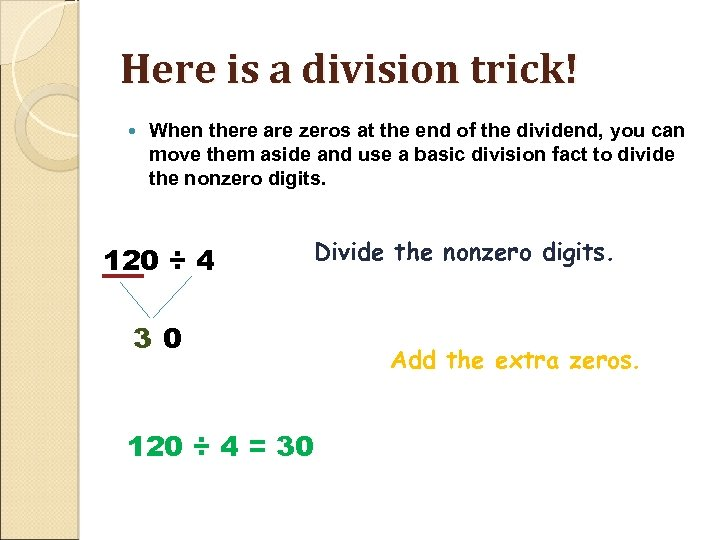Here is a division trick! When there are zeros at the end of the dividend, you can move them aside and use a basic division fact to divide the nonzero digits. 120 ÷ 4 3 0 120 ÷ 4 = 30 Divide the nonzero digits. Add the extra zeros.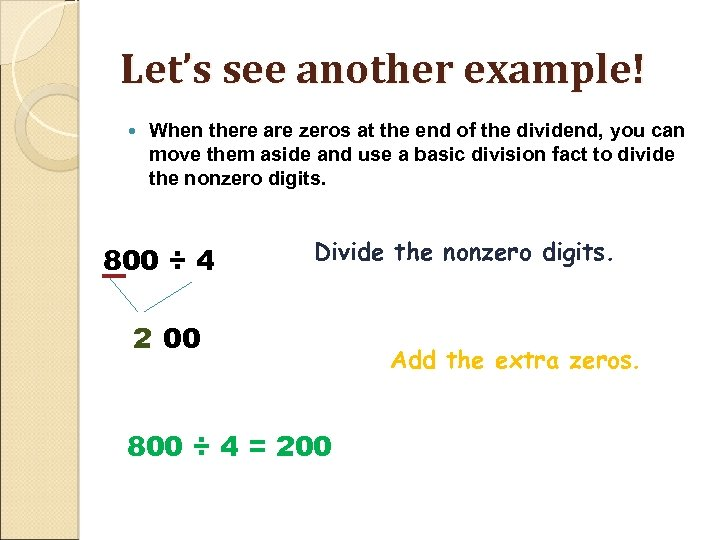Let’s see another example! When there are zeros at the end of the dividend, you can move them aside and use a basic division fact to divide the nonzero digits. 800 ÷ 4 Divide the nonzero digits. 2 00 800 ÷ 4 = 200 Add the extra zeros.Let’s see another example! When there are zeros at the end of the dividend, you can move them aside and use a basic division fact to divide the nonzero digits. 800 ÷ 4 Divide the nonzero digits. 2 00 800 ÷ 4 = 200 Add the extra zeros.Try some on your own! Solve the following problems in your Math notebook. Use place value patterns to help you! 3 x 100 = ____ 5 x 1, 000 = _____ 6 x 10 = ____ 18 x 100= ____ 2 x 1, 000 =____ 9 x 10 = _____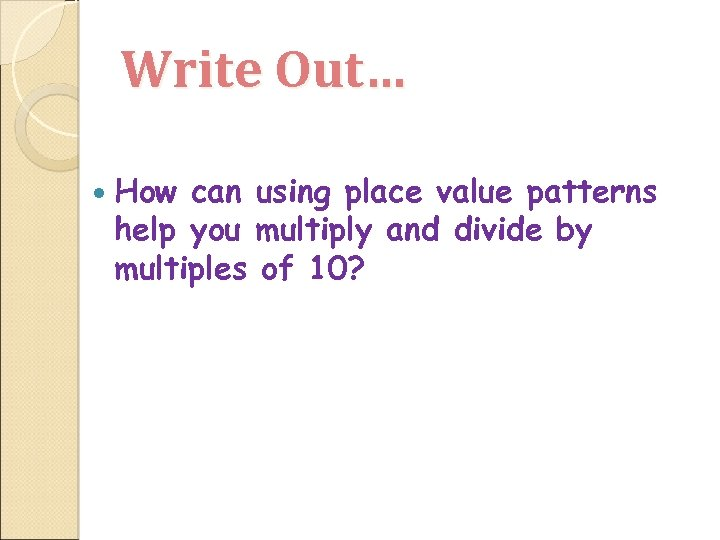Write Out… How can using place value patterns help you multiply and divide by multiples of 10?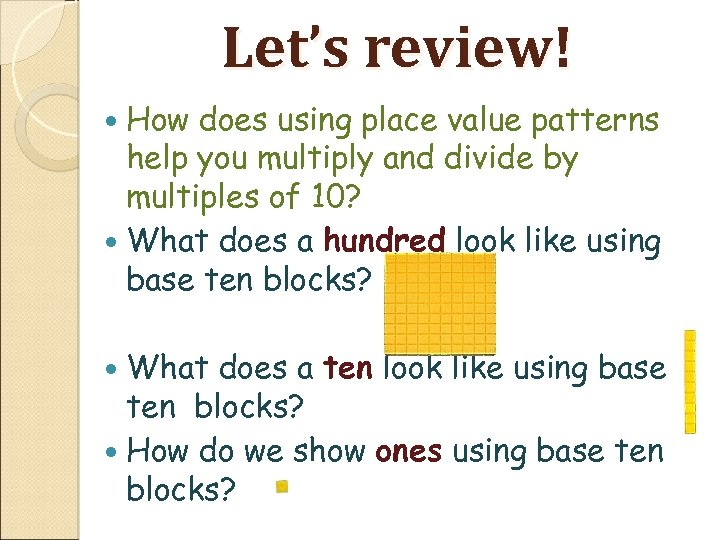Let’s review! How does using place value patterns help you multiply and divide by multiples of 10? What does a hundred look like using base ten blocks? What does a ten look like using base ten blocks? How do we show ones using base ten blocks?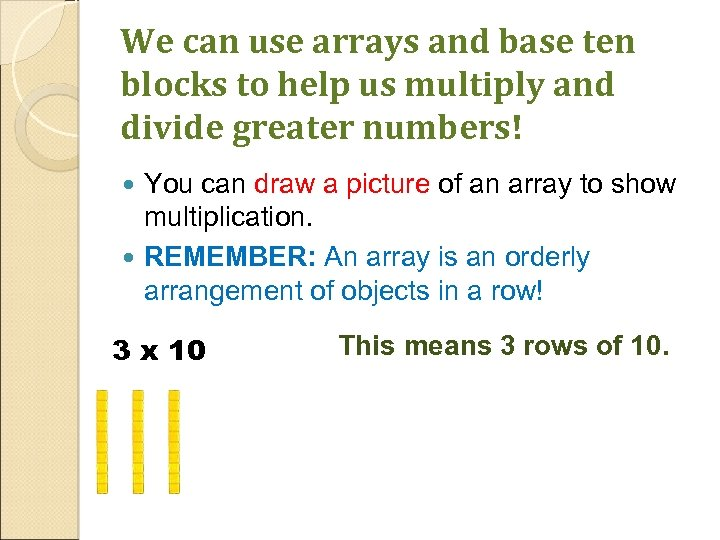We can use arrays and base ten blocks to help us multiply and divide greater numbers! You can draw a picture of an array to show multiplication. REMEMBER: An array is an orderly arrangement of objects in a row! 3 x 10 This means 3 rows of 10.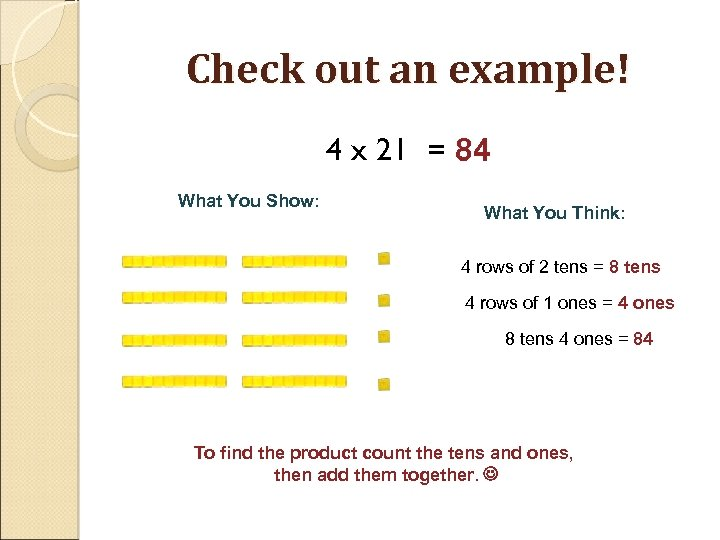Check out an example! 4 x 21 = 84 What You Show: What You Think: 4 rows of 2 tens = 8 tens 4 rows of 1 ones = 4 ones 8 tens 4 ones = 84 To find the product count the tens and ones, then add them together.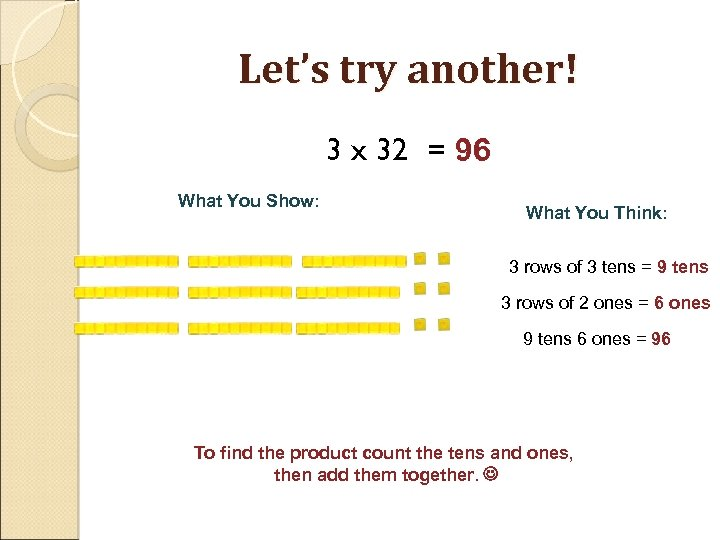Let’s try another! 3 x 32 = 96 What You Show: What You Think: 3 rows of 3 tens = 9 tens 3 rows of 2 ones = 6 ones 9 tens 6 ones = 96 To find the product count the tens and ones, then add them together.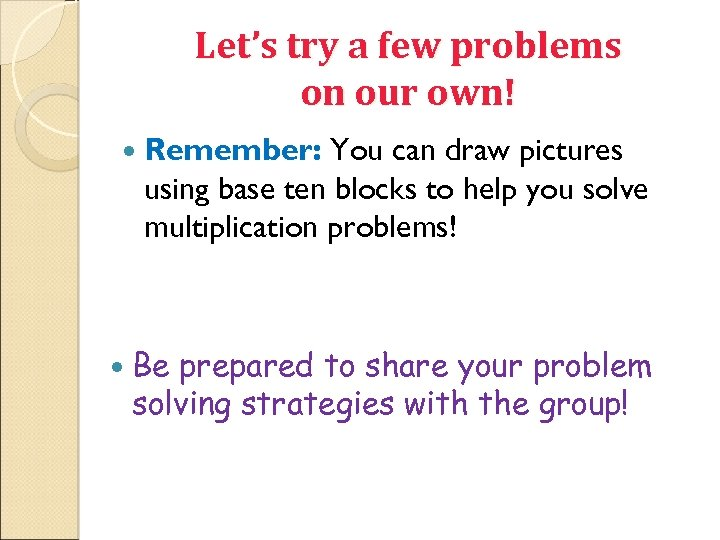Let’s try a few problems on our own! Remember: You can draw pictures using base ten blocks to help you solve multiplication problems! Be prepared to share your problem solving strategies with the group!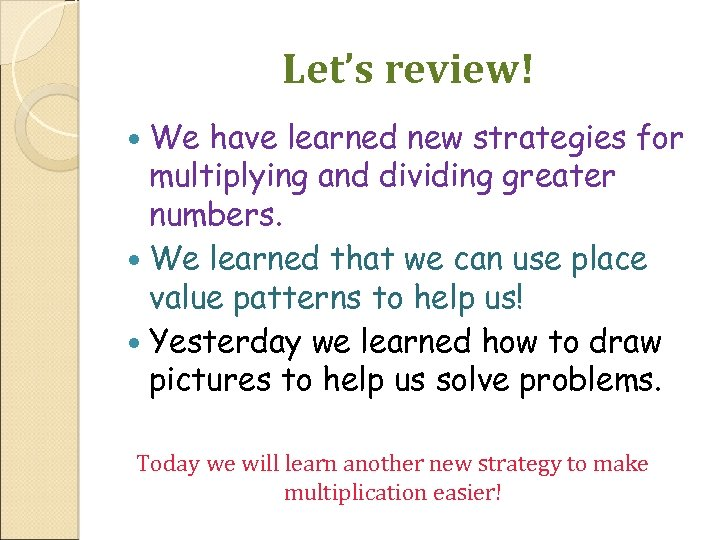Let’s review! We have learned new strategies for multiplying and dividing greater numbers. We learned that we can use place value patterns to help us! Yesterday we learned how to draw pictures to help us solve problems. Today we will learn another new strategy to make multiplication easier!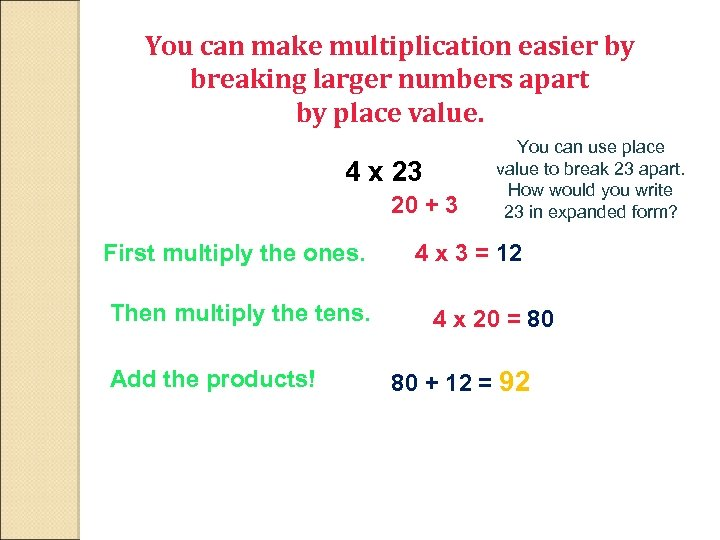You can make multiplication easier by breaking larger numbers apart by place value. 4 x 23 20 + 3 First multiply the ones. Then multiply the tens. Add the products! You can use place value to break 23 apart. How would you write 23 in expanded form? 4 x 3 = 12 4 x 20 = 80 80 + 12 = 92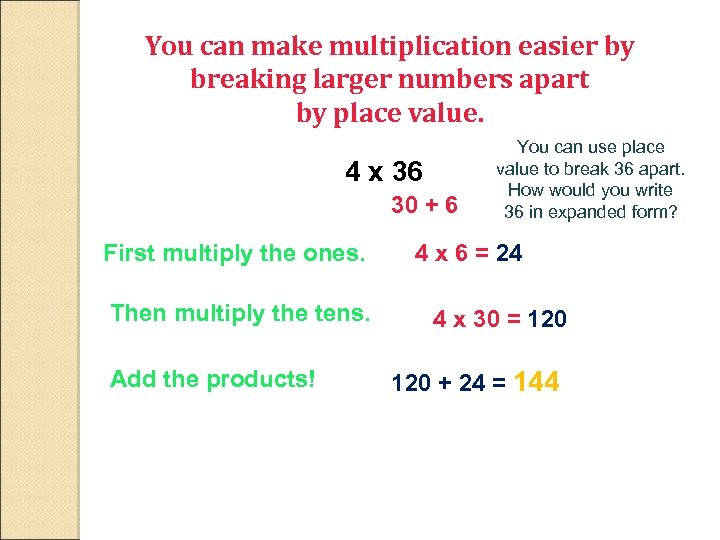You can make multiplication easier by breaking larger numbers apart by place value. 4 x 36 30 + 6 First multiply the ones. Then multiply the tens. Add the products! You can use place value to break 36 apart. How would you write 36 in expanded form? 4 x 6 = 24 4 x 30 = 120 + 24 = 144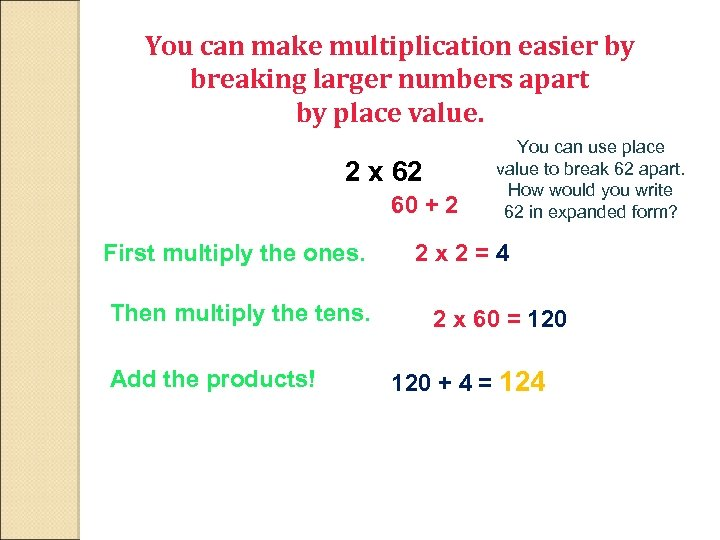You can make multiplication easier by breaking larger numbers apart by place value. 2 x 62 60 + 2 First multiply the ones. Then multiply the tens. Add the products! You can use place value to break 62 apart. How would you write 62 in expanded form? 2 x 2=4 2 x 60 = 120 + 4 = 124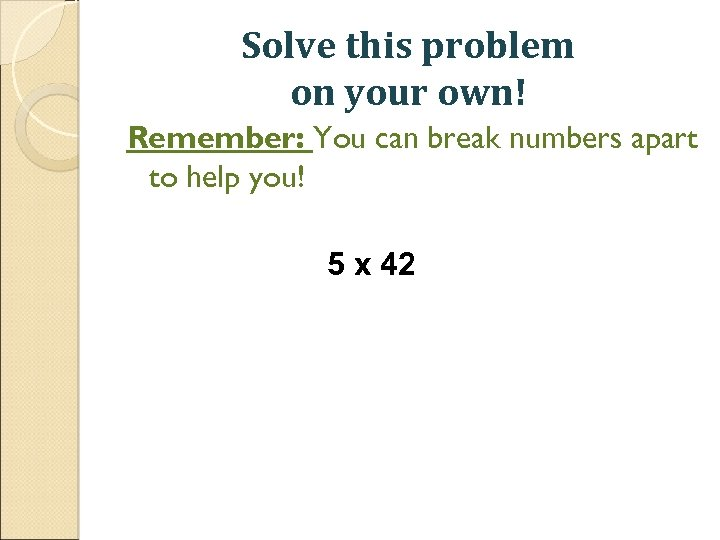Solve this problem on your own! Remember: You can break numbers apart to help you! 5 x 42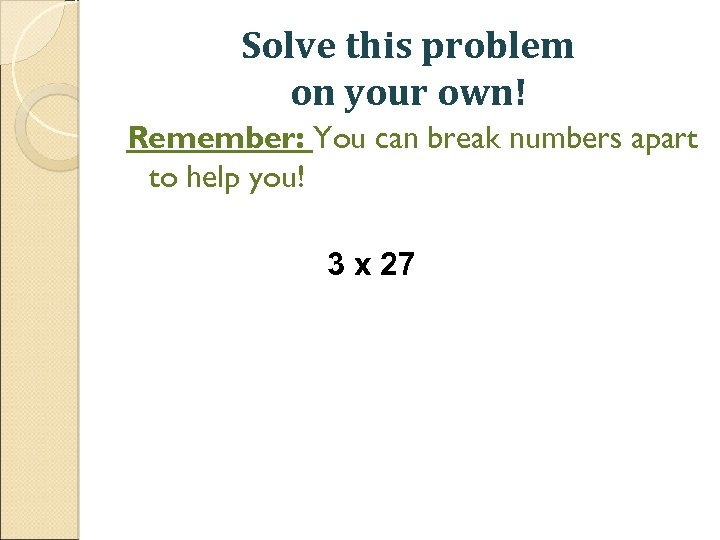Solve this problem on your own! Remember: You can break numbers apart to help you! 3 x 27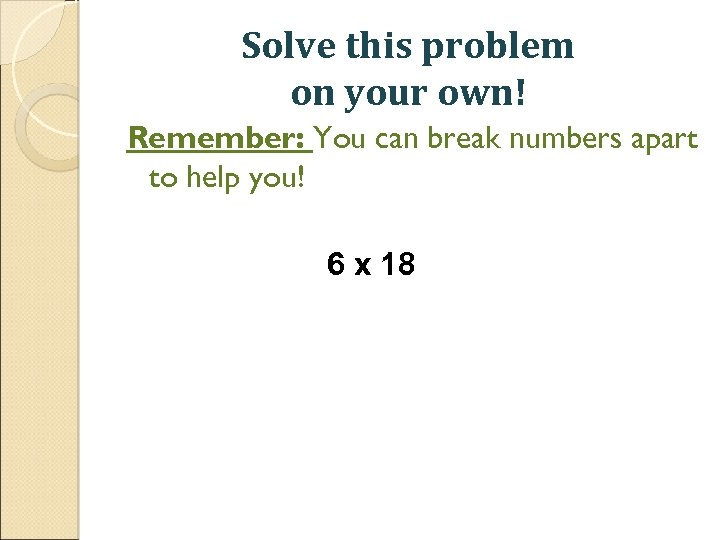Solve this problem on your own! Remember: You can break numbers apart to help you! 6 x 18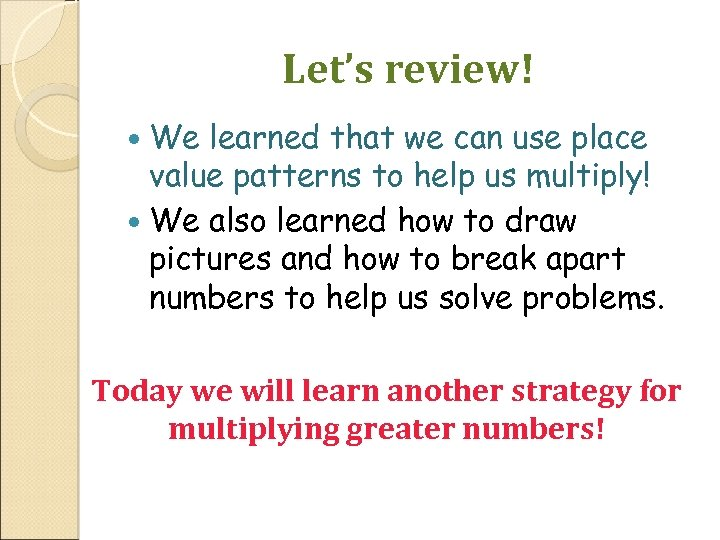Let’s review! We learned that we can use place value patterns to help us multiply! We also learned how to draw pictures and how to break apart numbers to help us solve problems. Today we will learn another strategy for multiplying greater numbers!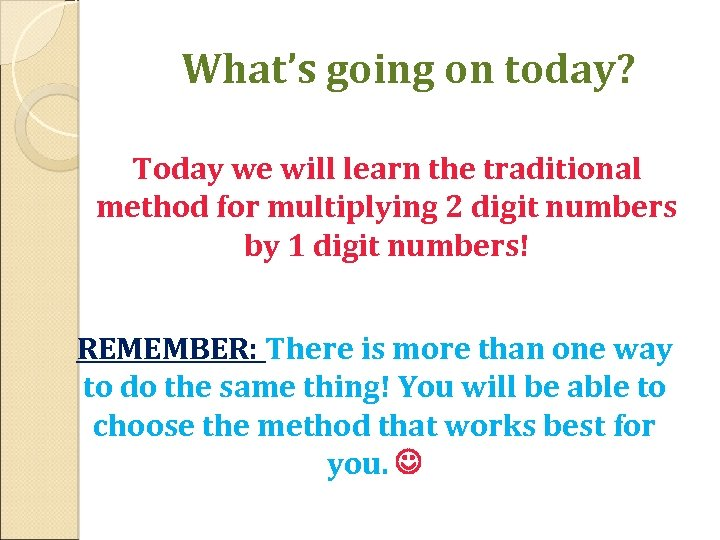What’s going on today? Today we will learn the traditional method for multiplying 2 digit numbers by 1 digit numbers! REMEMBER: There is more than one way to do the same thing! You will be able to choose the method that works best for you.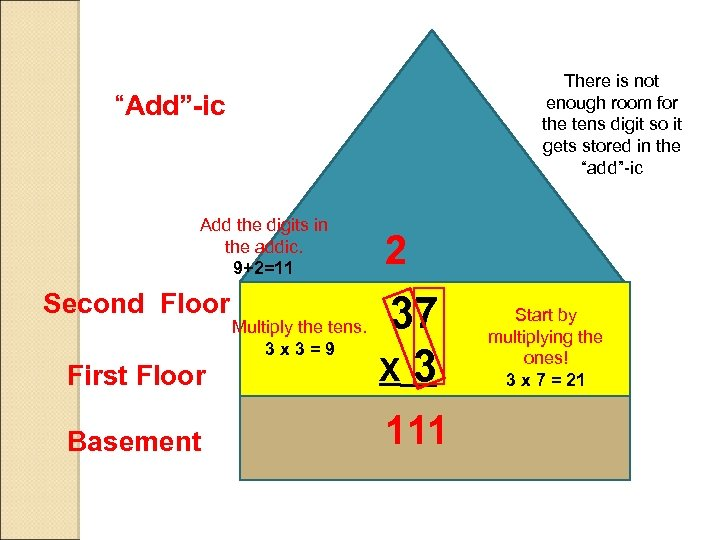There is not enough room for the tens digit so it gets stored in the “add”-ic “Add”-ic Add the digits in the addic. 9+2=11 Second Floor 2 First Floor 37 X 3 Basement 111 Multiply the tens. 3 x 3=9 Start by multiplying the ones! 3 x 7 = 21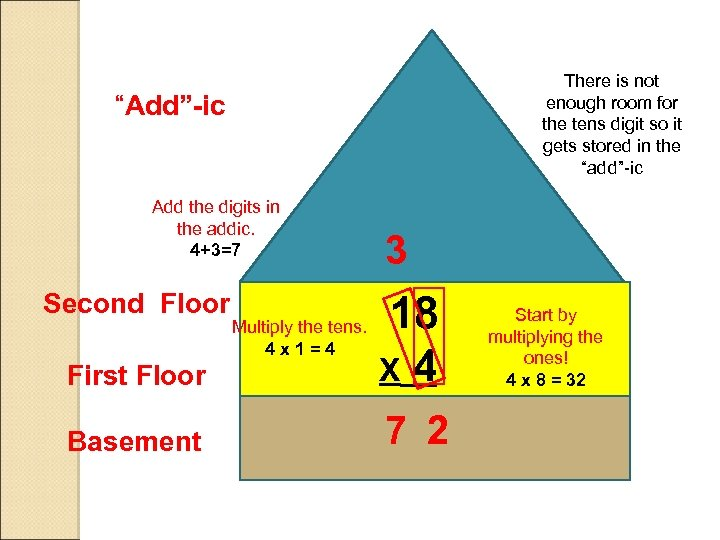There is not enough room for the tens digit so it gets stored in the “add”-ic “Add”-ic Add the digits in the addic. 4+3=7 Second Floor 3 First Floor 18 X 4 Basement 7 2 Multiply the tens. 4 x 1=4 Start by multiplying the ones! 4 x 8 = 32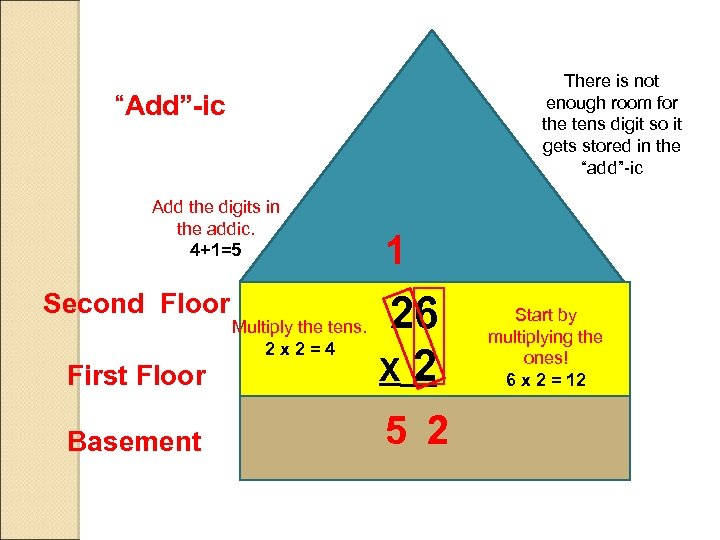There is not enough room for the tens digit so it gets stored in the “add”-ic “Add”-ic Add the digits in the addic. 4+1=5 Second Floor 1 First Floor 26 X 2 Basement 5 2 Multiply the tens. 2 x 2=4 Start by multiplying the ones! 6 x 2 = 12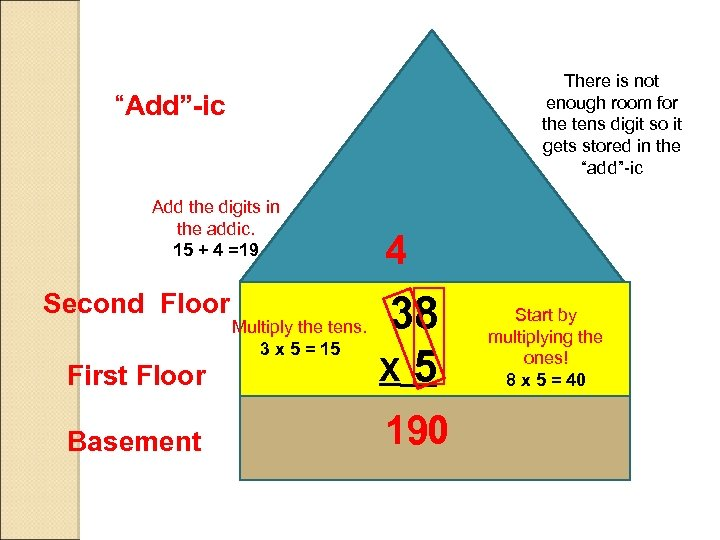There is not enough room for the tens digit so it gets stored in the “add”-ic “Add”-ic Add the digits in the addic. 15 + 4 =19 Second Floor 4 First Floor 38 X 5 Basement 190 Multiply the tens. 3 x 5 = 15 Start by multiplying the ones! 8 x 5 = 40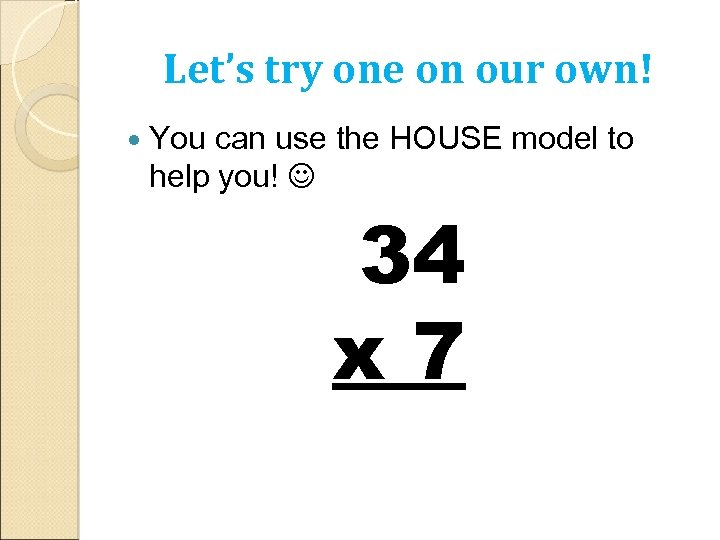Let’s try one on our own! You can use the HOUSE model to help you! 34 x 7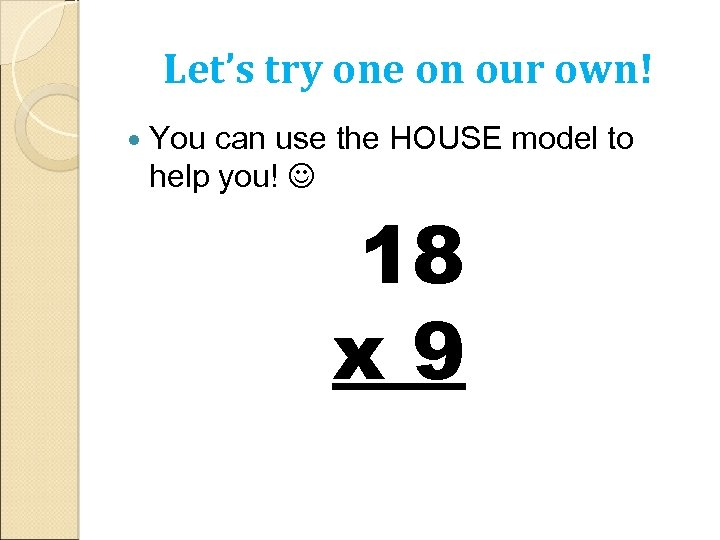Let’s try one on our own! You can use the HOUSE model to help you! 18 x 9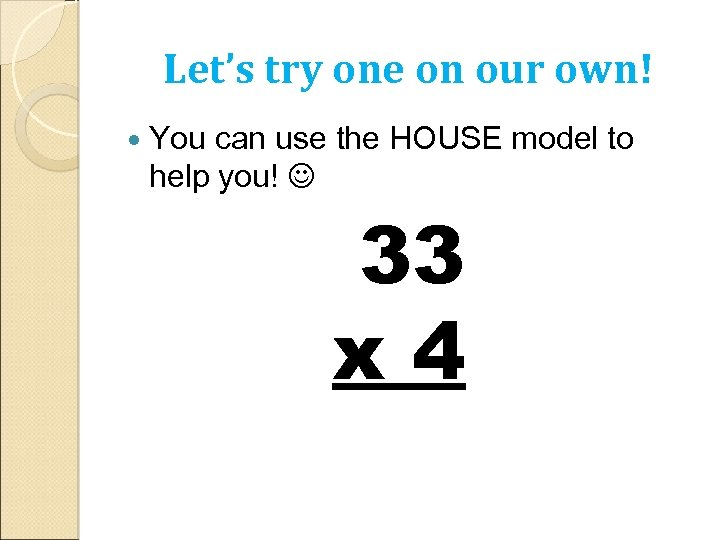Let’s try one on our own! You can use the HOUSE model to help you! 33 x 4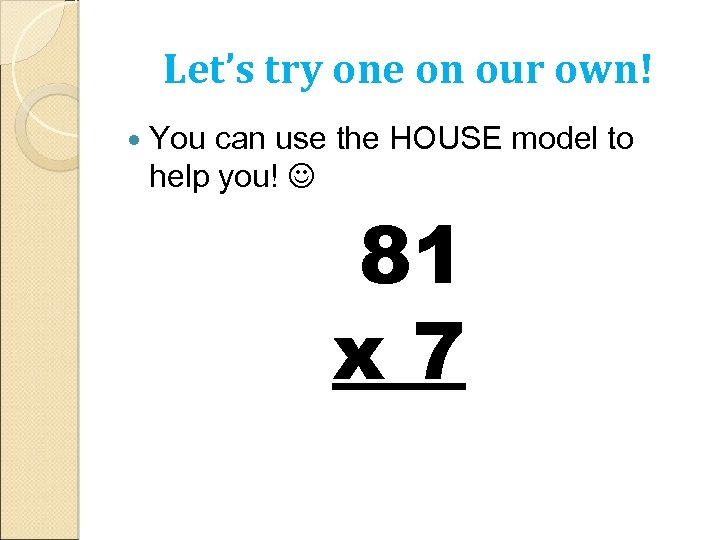Let’s try one on our own! You can use the HOUSE model to help you! 81 x 7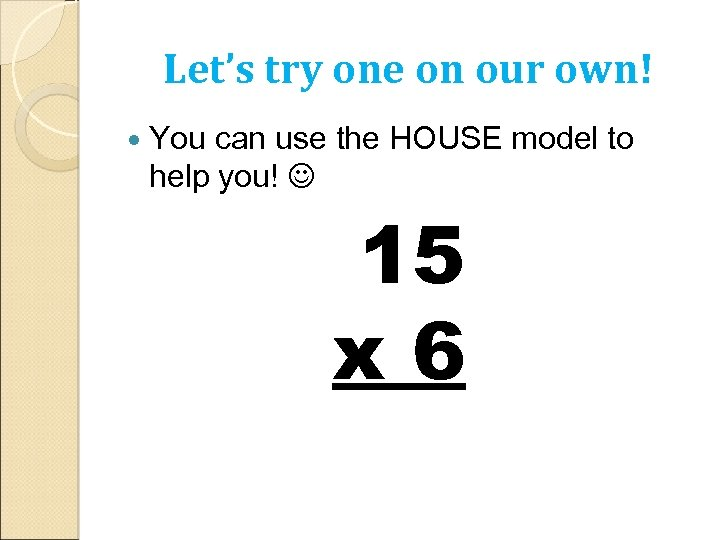Let’s try one on our own! You can use the HOUSE model to help you! 15 x 6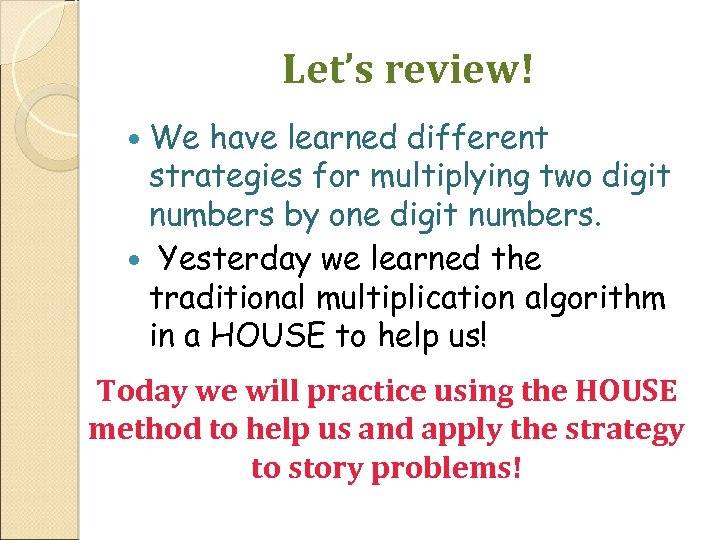Let’s review! We have learned different strategies for multiplying two digit numbers by one digit numbers. Yesterday we learned the traditional multiplication algorithm in a HOUSE to help us! Today we will practice using the HOUSE method to help us and apply the strategy to story problems!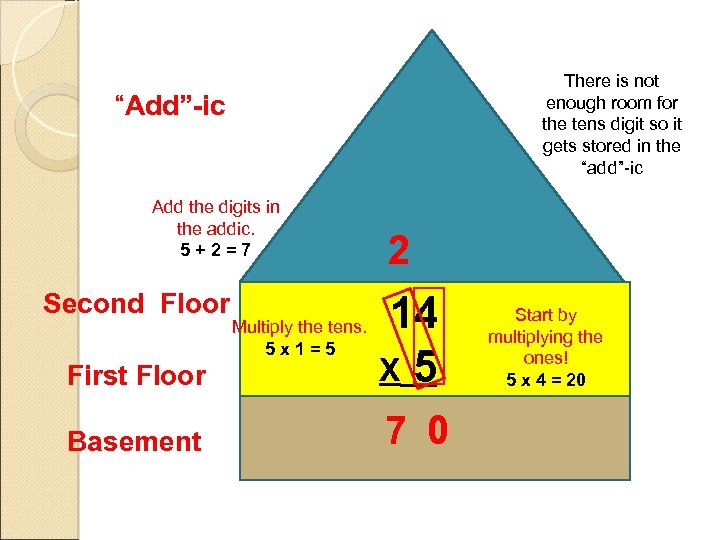There is not enough room for the tens digit so it gets stored in the “add”-ic “Add”-ic Add the digits in the addic. 5+2=7 Second Floor 2 First Floor 14 X 5 Basement 7 0 Multiply the tens. 5 x 1=5 Start by multiplying the ones! 5 x 4 = 20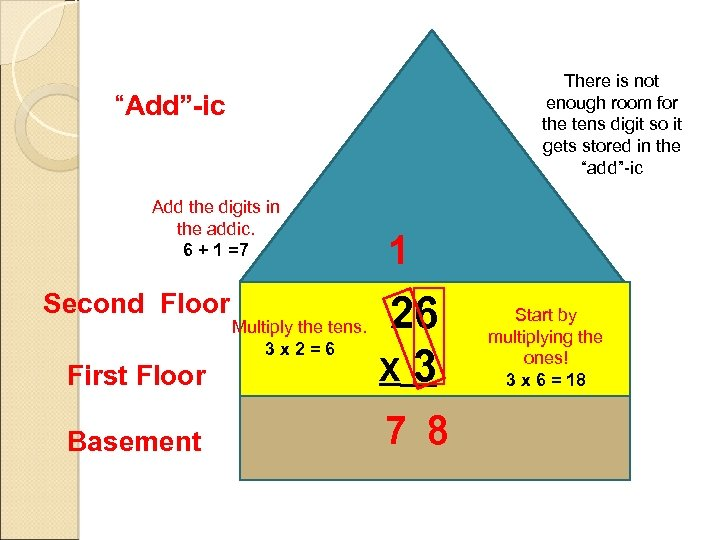There is not enough room for the tens digit so it gets stored in the “add”-ic “Add”-ic Add the digits in the addic. 6 + 1 =7 Second Floor 1 First Floor 26 X 3 Basement 7 8 Multiply the tens. 3 x 2=6 Start by multiplying the ones! 3 x 6 = 18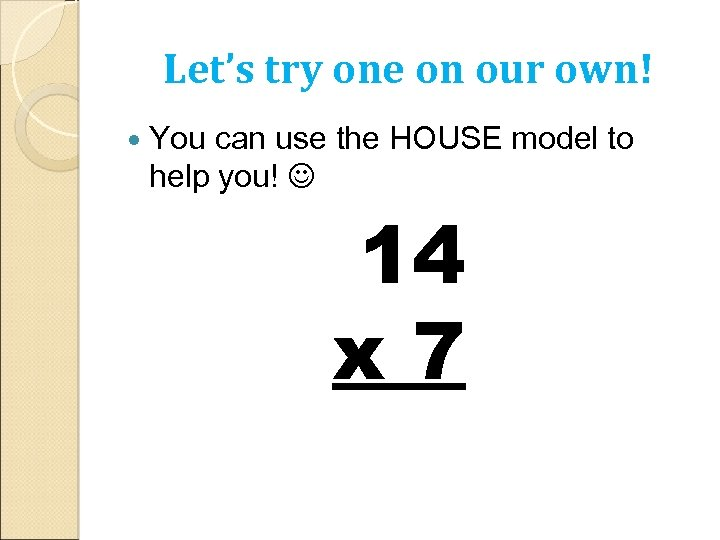Let’s try one on our own! You can use the HOUSE model to help you! 14 x 7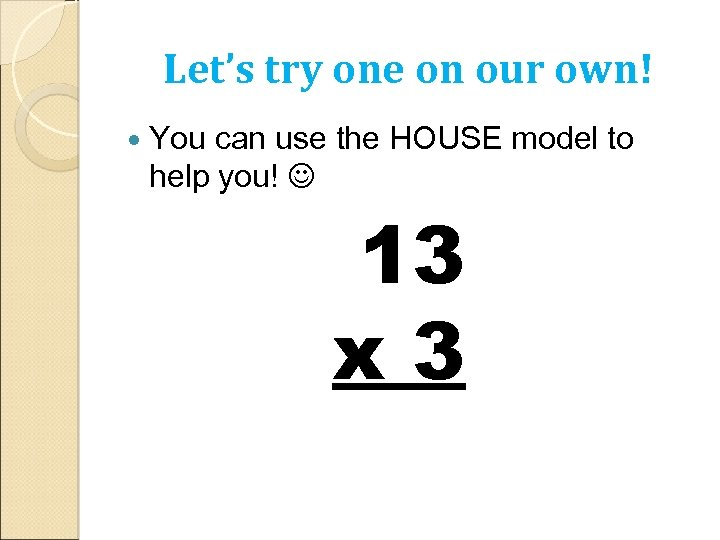Let’s try one on our own! You can use the HOUSE model to help you! 13 x 3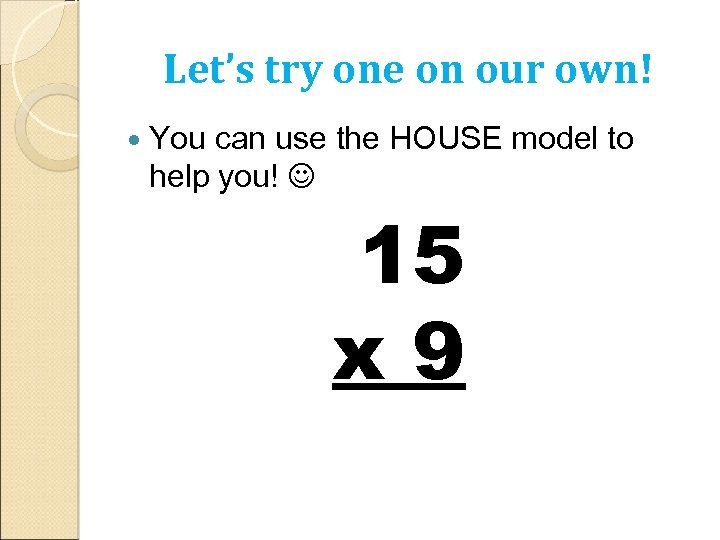Let’s try one on our own! You can use the HOUSE model to help you! 15 x 9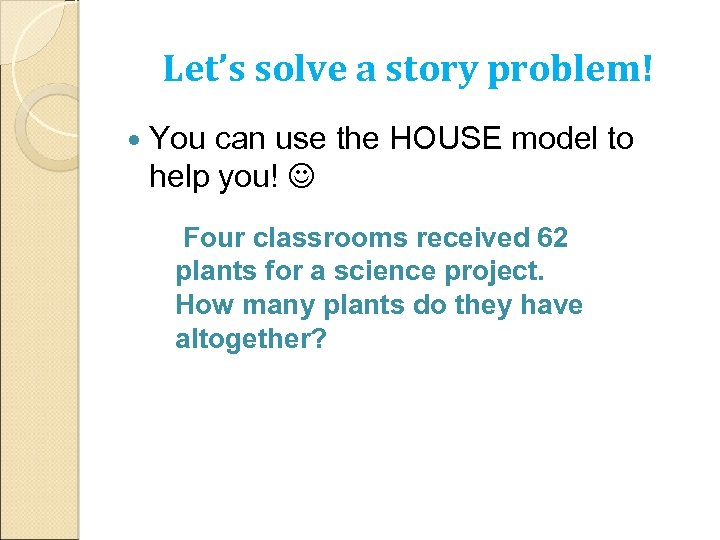Let’s solve a story problem! You can use the HOUSE model to help you! Four classrooms received 62 plants for a science project. How many plants do they have altogether?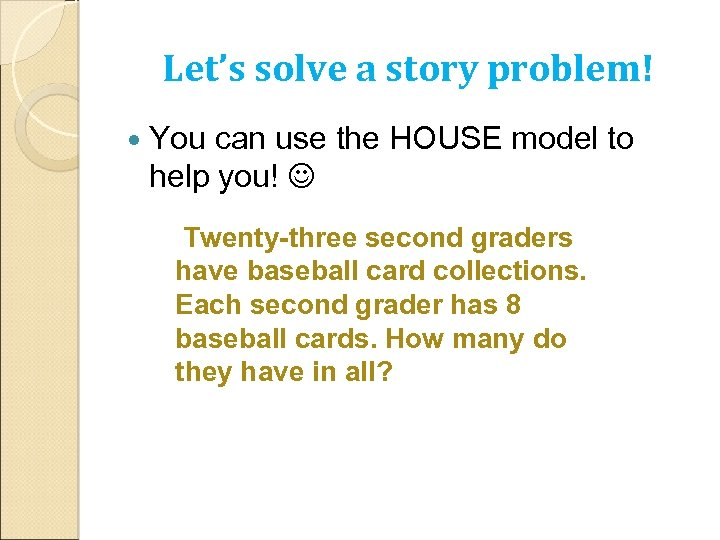Let’s solve a story problem! You can use the HOUSE model to help you! Twenty-three second graders have baseball card collections. Each second grader has 8 baseball cards. How many do they have in all?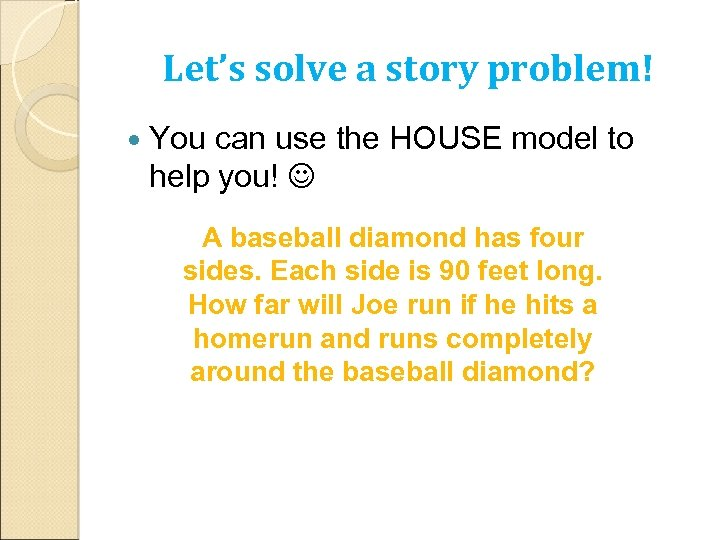Let’s solve a story problem! You can use the HOUSE model to help you! A baseball diamond has four sides. Each side is 90 feet long. How far will Joe run if he hits a homerun and runs completely around the baseball diamond?